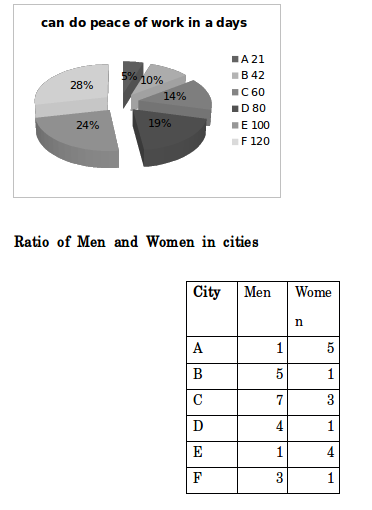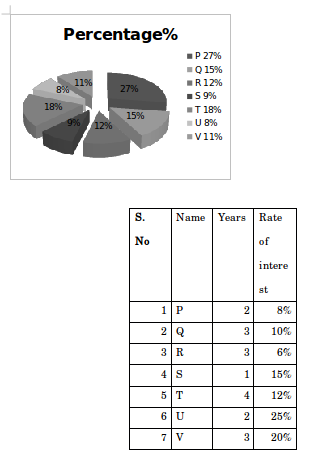## Important Data Interpretation For SBI PO – Set – 68

D.1-5) Study the following information carefully and answer the questions given bellow:1) If city C completed a piece of work costing Rs.3500. What is the wage of Men’s from city C?

a) 2250

b) 2450

c) 2550

d) 2650

e) None of these

b)

C can do peace of work=60 day’s

M:F=7:3

According to question,

7 Men’ s can do alone in 60×7/10=42 days

3 Women’ s can do alone in 60×3/10=18 days

Men’ s wages=3500

=>using crossing multiplication

3500×42=60 ×x =>x=2450

2) For completing a certain work a wages of 9500 has been fixed. If Men in city D and Women in city E tends to do the job. What is the wage of Women from city E (Approximately),

a) Rs.2318

b) Rs.3318

c) Rs.4318

d) Rs.5318

e) None of these

c)

D’s can do peace of work=80 day’ s

M:F=4:1

E’s can do peace of work=100 day’ s

M:F=1:4

According to question,

Men can do alone in D=80×5/4=100 day’s

Woman can do alone in E=100×5/4=120 day’s

Require answer, (Efficiency of D and E)

D/E=((1/100))/(1/120) =>D/E=120/100=6/5

Wage of women from city E,

9500×5/11=4318.18~ 4318

3) A and B begin together but 3 days before the completion of the work, A leaves off. The total number of days for the work to be completed is

a) 13 days

b) 14 days

c) 15 days

d) 16 days

e) None of these

d

B’s three day’s work=3×1/42=1/14; Remaining work=1-1/14=13/14.

(A+B)’s one day’s work=1/21+1/42=3/42=1/14.

13/14 work in finished by (A+B) in (13/14×14/1) days=13 days.

Total number of days=3+13=16 days.

16 days.

4) D, E and F completed a piece of work costing Rs.13172. If their daily wages are in the ratio 5:6:4, How much amount will be received by Men in F?

a) Rs.968

b) Rs.1182

c) Rs.1168

d) Rs.1268

e) None of these

b)

According to question,

Ratio of wages of D,E,F=(80×5) :(100×6) :(120×4)=400 :600 :480=10 :15 :12

F’s share=(13172 ×12/37)=Rs. 4272

Men in E’s share=(4272×3/4)=Rs. 1181.75~1182

5) A and B work together for 6 days and the rest of the work is done by C in 2 days. If they get Rs.4200 for the whole work, How much amount will be received by Women in C?

a) Rs.720

b) Rs.800

c) Rs.840

d) Rs.820

e) None of these

a)

(A+B)’ s six day’s work=6(1/21+1/42)=6(3/42)=6/14=3/7

Remaining work=1-3/7=4/7.

C’ s two days work=4/7

=(A’s six days’ work) :(B’ s six days’ work) :(C’ s two day s work)

=6/21 :6/42 :4/7=2/7 :1/7 :4/7 =>2 :1 :4

C’s share=(4200×4/7)=Rs. 2400

=2400×3/10=Rs.720

D.6-10) Study the following information carefully and answer the questions given below:Total monthly amount of principal = Rs.10,0006) What is the difference between the difference of simple and compound interest to Q and the difference of simple and compound interest to P in given years?

a) Rs.250.64

b) Rs.350.64

c) Rs.450.64

d) Rs.550.64

e) None of these

b)

Sum of principal amount P per annum=10000×27/100×12=32400

Sum of principal amount Q per annum=10000×15/100×12=18000

According to question,

The difference of simple and compound interest to P=32400(8/100)^2=207.36

The difference of simple and compound interest to Q=18000 (10/100)^2 ((300+10)/100)=558

Q-P=558-207.36=350.64

7) Find compound interest for 6 months compounded quarterly in T?

a) Rs.2569.76

b) Rs.2669.76

c)Rs.2769.76

d) Rs.2869.76

e) None of these

b)

Principle amount of T per annum=10000×18/100×12=21600

R=6% per half-year, t=2 half-years

Amount={21600(1+6/100)^2 }=21600×53/50×53/50=24269.76

CI=(24269.76-21600)=Rs. 2669.76

8) What is the total amount to T in given years? (using Simple interest)

a) Rs.28240

b) Rs.29240

c) Rs.31968

d) Rs.31240

e) None of these

c)

According to question,

Toal amount of T=21600 (1+(4×12)/100)=31968

9) What is the respective ratio of the total amount of U to the total amount of V with using compound interest? (Approximately)

a) 422 : 625

b) 950 : 625

c) 820 : 387

d) 625 : 950

e) None of these

d)

According to question,

The total amount of U,

Principal=10000×8/100×12=9600

U=9600((1+25/100)^2 )=Rs.15000

The total amount of V,

principle=10000×11/100×12=13200

V=13200((1+20/100)^3 )=22809.6

U :V=15000 :22809.6

U :V=625 :950.4 ~ 625 :950

10) What is the average of the total amount of P, Q, R and S using simple interest in given years?

a) Rs.22599

b) Rs.23599

c) Rs.24599

d) Rs.25599

e) None of these

a)

According to question,

Principal of amount P=10000×27/100×12=32400

Principal of amount Q=10000×15/100×12=18000

Principal of amount R=10000×12/100×12=14400

Principal of amount S=10000×9/100×12=10800

Total amount of P=32400(1+(2×8)/100)=37584

Total amount of Q=18000(1+(3×10)/100)=23400

Total amount of R=14400(1+(3×6)/100)=16992

Total amount of S=10800 (1+(1×15)/100)=12420